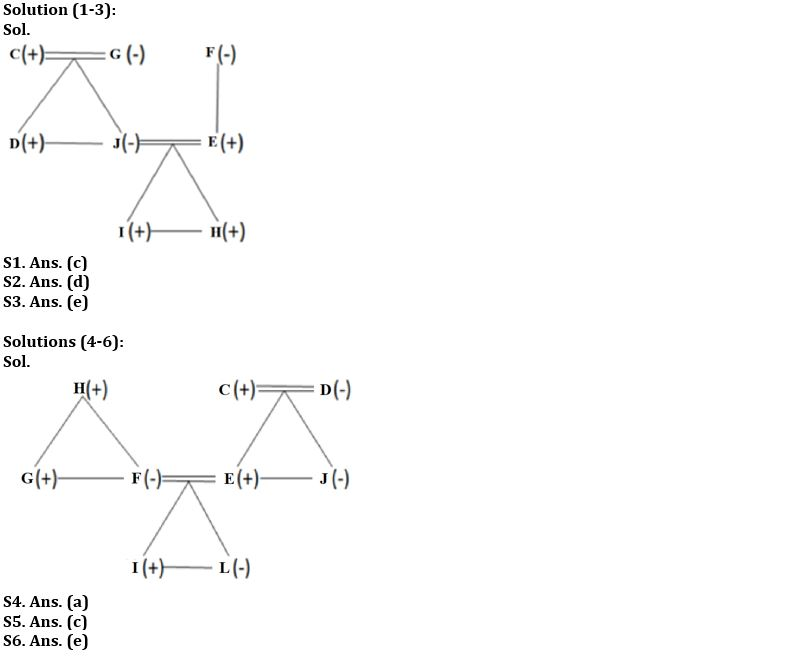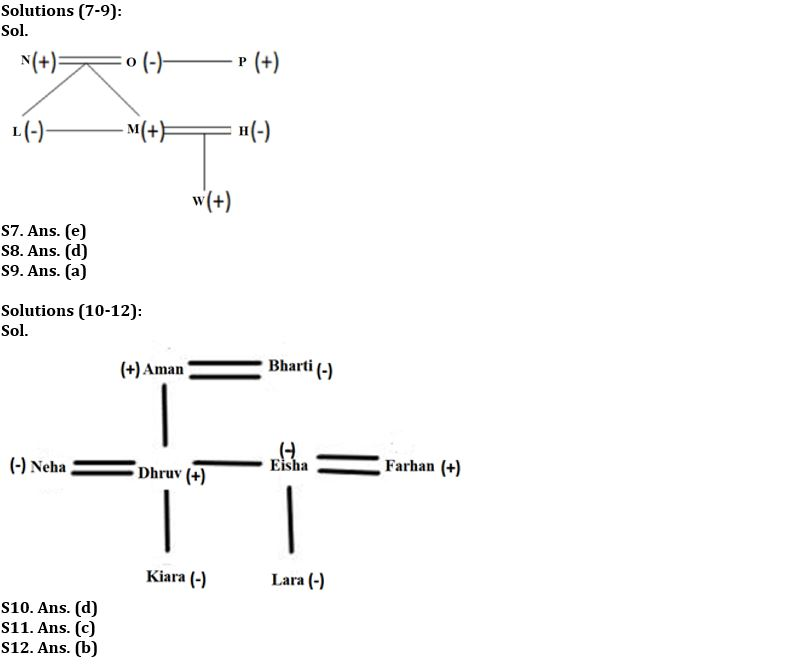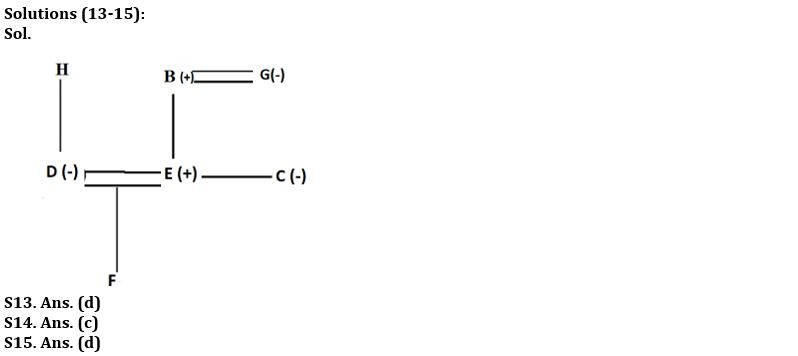Latest Banking jobs   »

# Reasoning Ability Quiz For FCI Phase I 2023- 6th January

Direction (1-3): Study the data carefully and answer the questions accordingly.
There are eight members in a three generations family. There are two married couples. C is the father of D who is the brother-in-law of E. G has a son and a daughter. H and I are the grandsons of F. G is the mother-in-law of E. There are at most five males. J is the daughter-in-law of F who has only one child. E is the son of F.

Q1. How many females are there?
(a) Two
(b) Five
(c) Three
(d) Four
(e) None of these

Q2. How C is related to I?
(a) Uncle
(b) Nephew
(c) Son
(d) Grandfather
(e) None of these

Q3. Four from the following are similar in a certain way and forms a group. Find out the one who does not belong to that group.
(a) I
(b) H
(c) C
(d) D
(e) G

Direction (4-6): Study the data carefully and answer the questions accordingly.
There are eight members in a family with two married couples and three generations. C is the grandfather of I who is the nephew of J. L is the daughter of F. E is the brother-in-law of G who is unmarried. H is the father of F. There are four females. D is the mother of J who is the sister-in law of F. J is unmarried. C has only one daughter.

Q4. How D is related to L?
(a) Grandmother
(b) Sister
(c) Aunt
(d) Mother
(e) None of these

Q5. How J is related to I?
(a) Niece
(b) Mother
(c) Aunt
(d) Sister
(e) None of these

Q6. Find the correct statement from the following.
(a) H is the father of J
(b) I and L are not siblings
(c) F is the sister-in-law of G
(d) C is the father of G
(e) None is correct

Direction (7-9): Study the data carefully and answer the questions accordingly.
There are seven members in a family with two married couples. N is the grandfather of W who is the son of H. L and M are the children of O who is the sister of P. L is an unmarried female. Number of males is more than the number of females. H is sister-in-law of L.

Q7. How P is related to N?
(a) Uncle
(b) Nephew
(c) Brother
(d) Son
(e) Brother-in-law

Q8. How N is related to H?
(a) Bother
(b) Uncle
(c) Father
(d) Father-in-law
(e) Son

Q9. Who among the following is the married couple?
(a) N and O
(b) L and M
(c) O and P
(d) N and L
(e) None of the above

Direction (10-12): Study the following information carefully and answer the questions given below.
There are three married couples in the family. Dhruv is the only son of Aman. Eisha is the only sister-in-law of Neha. Bharti is the grandmother of Kiara who is the daughter of Dhruv. Farhaan is the father of Lara who is the only daughter of Eisha. Bharati is mother-in-law of Neha.

Q10. How is Kiara related to Lara?
(a) Brother
(b) Sister
(c) Mother
(d) Cousin
(e) None of these

Q11. How many males are there in the family?
(a) One
(b) Two
(c) Three
(d) Four
(e) Five

Q12. If Charan is the brother of Bharti, then how is Charan related to Dhruv?
(a) Father
(b) Maternal Uncle
(c) Brother
(d) Son
(e) None of these

Direction (13-15): Study the following information and answer the given questions.
In a family of three generations, there are seven family members B, C, D, E, F, G and H. B is the father of E and C. D and E are a married couple, D is the only child of H. D has no brother-in-law. F is the child of E. G is the mother-in-law of D and D is the sister-in-law of C, there are two married couples in a family.

Q13. How is H related to E?
(a) Grandson
(b) Mother-in- law
(c) Father -in- law
(d) Cannot be Determined
(e) None of the above

Q14. Who is the father of F?
(a) D
(b) G
(c) E
(d) H
(e) None of the above

Q15. How many male members are there in the family?
(a) One
(b) Two
(c) Three
(d) Cannot be determined
(e) None of the above

Solutions## FAQs

### Q1. How many females are there? (a) Two (b) Five (c) Three (d) Four (e) None of these

S1. Ans (c)

#### Congratulations!